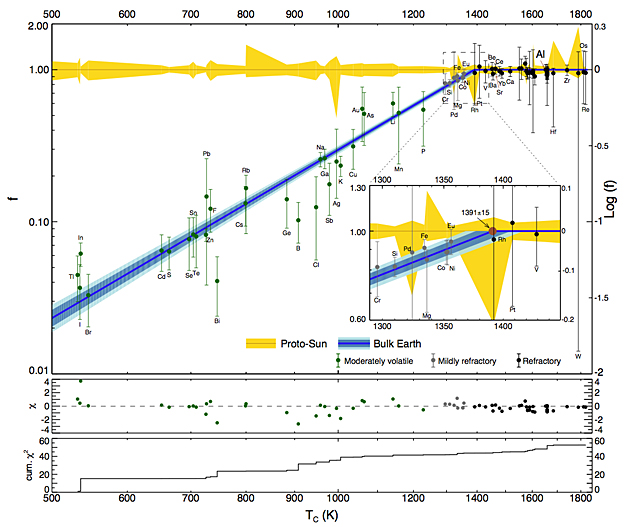# The Volatility Trend of Protosolar and Terrestrial Elemental AbundancesComparison of the elemental abundances of the Earth and Sun. This plot is similar to Fig. 4 except here, we only plot elements with TC > 500 K and the TC axis is logarithmic. The diagonal dark blue line and its horizontal continuation in the upper right, is our best χ2 fit to the data. The dark and light blue diagonal regions are the 68% and 95% confidence intervals on the fit. For details see Appendix C. The diagonal dark blue line reaches f = 1 at the critical devolatilization temperature TD(E) = 1391 ± 15 K. The inset shows more of the details of this region. The lower two panels show the values of χ and the cumulative χ2 as a function of TC .

We present new estimates of protosolar elemental abundances based on an improved combination of solar photospheric abundances and CI chondritic abundances. These new estimates indicate CI chondrites and solar abundances are consistent for 60 elements.

Our estimate of the protosolar "metallicity" (i.e. mass fraction of metals, Z) is 1.40%, which is consistent with a value of Z that has been decreasing steadily over the past three decades from ∼ 1.9%. We compare our new protosolar abundances with our recent estimates of bulk Earth composition (normalized to aluminium), thereby quantifying the devolatilization in going from the solar nebula to the formation of the Earth. The quantification yields a linear trend log( f ) = α log(TC ) + β, where f is the Earth-to-Sun abundance ratio and TC is the 50% condensation temperature of elements. The best fit coefficients are: α = 3.676 ± 0.142 and β = −11.556 ± 0.436. The quantification of these parameters constrains models of devolatilization processes. For example, the coefficients α and β determine a critical devolatilization temperature for the Earth TD(E) = 1391 ± 15 K. The terrestrial abundances of elements with TC < TD(E) are depleted compared with solar abundances, whereas the terrestrial abundances of elements with TC > TD(E) are indistinguishable from solar abundances.

The abundances of noble gases and hydrogen are depleted more than a prediction based on the extrapolation of the best-fit volatility trend. The terrestrial abundance of Hg (TC = 252 K) appears anomalously high under the assumption that solar and CI chondrite Hg abundances are identical. To resolve this anomaly, we propose that CI chondrites have been depleted in Hg relative to the Sun by a factor of 13 ± 7. We use the best-fit volatility trend to derive the fractional distribution of carbon and oxygen between volatile and refractory components ( fvol , fref ). For carbon we find (0.91 ± 0.08, 0.09 ± 0.08); for oxygen we find (0.80 ± 0.04, 0.20 ± 0.04). Our preliminary estimate gives CI chondrites a critical devolatilization temperature TD(CI) = 550 +20/−100 K.

Haiyang S. Wang, Charles H. Lineweaver, Trevor R. Ireland
(Submitted on 30 Oct 2018)